# What is Blocked Rotor Test of an Induction Motor?

Posted on

What is blocked rotor test of an induction motor? A blocked rotor test is normally performed on an induction motor to find out the leakage impedance. Apart from it, other parameters such as torque, motor, short-circuit current at normal voltage, and many more could be found from this test. Blocked rotor test is analogous to the short circuit test of transformer.

Here shaft of the motor is clamped i.e. blocked so it cannot move and rotor winding is short circuited. In slip ring motor rotor winding is short circuited through slip rings and in cage motors, rotors bars are permanently short circuited.

# What is Blocked Rotor Test of an Induction Motor?

The testing of the induction motor is a little bit complex as the resultant value of leakage impedance may get affected by rotor position, rotor frequency and by magnetic dispersion of the leakage flux path. These effects could be minimized by conducting a block rotor current test on squirrel-cage rotors.

## Process of Blocked Rotor Test of an Induction Motor

In the blocked rotor test, it should be kept in mind that the applied voltage on the stator terminals should be low otherwise normal voltage could damage the winding of the stator.

In block rotor test, the low voltage is applied so that the rotor does not rotate and its speed becomes zero and full load current passes through the stator winding.

The slip is unity related to zero speed of rotor hence the load resistance becomes zero. Now, slowly increase the voltage in the stator winding so that current reaches to its rated value.

At this point, note down the readings of the voltmeter, wattmeter and ammeter to know the values of voltage, power and current. The test can be repeated at different stator voltages for the accurate value.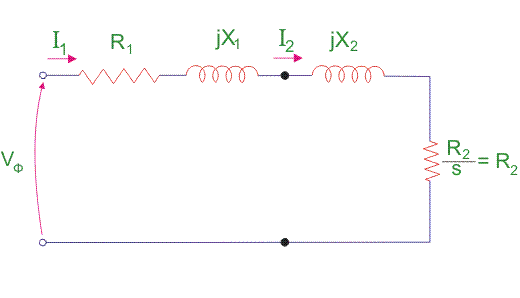## Calculations of Blocked Rotor Test of an Induction Motor

### Resistance and Leakage Reactance Values

In blocked rotor test, core loss is very low due to the supply of low voltage and frictional loss is also negligible as rotor is stationary, but stator cupper losses and the rotor cupper losses are reasonably high.Let us take denote copper loss by Wcu.

Therefore,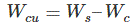Where, Wc = core loss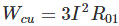Where, R01 = Motor winding of stator and rotor as per phase referred to stator.

Thus,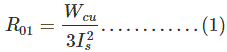Now let us consider

• Is = short circuit current
• Vs = short circuit voltage
• Z0 = short circuit impedance as referred to stator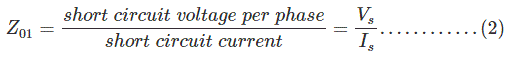Therefore,

X01 = Motor leakage reactance per phase referred to stator can be calculated as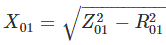Stator reactance X1 and rotor reactance per phase referred to stator X2 are normally assumed equal.

Therefore,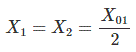Similarly, stator resistance per phase R1 and rotor resistance per phase referred to stator R2 can be calculated as follows:

First some suitable test are done on stator windings to find the value of R1 and then to find R2 subtract the R1 from R01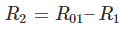### Short Circuit Current for Normal Supply Voltage

To calculate short circuit current Isc at normal voltage V of the stator, we must note short-circuit current Is and low voltage Vs applied to the stator winding.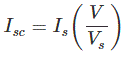### Blocked Rotor Test of an Induction Motor Conclusion

After going through the above portion of blocked rotor test of induction motor we can now establish calculations blocked rotor test of induction motor. I hope you enjoy when reading this article, thank you.